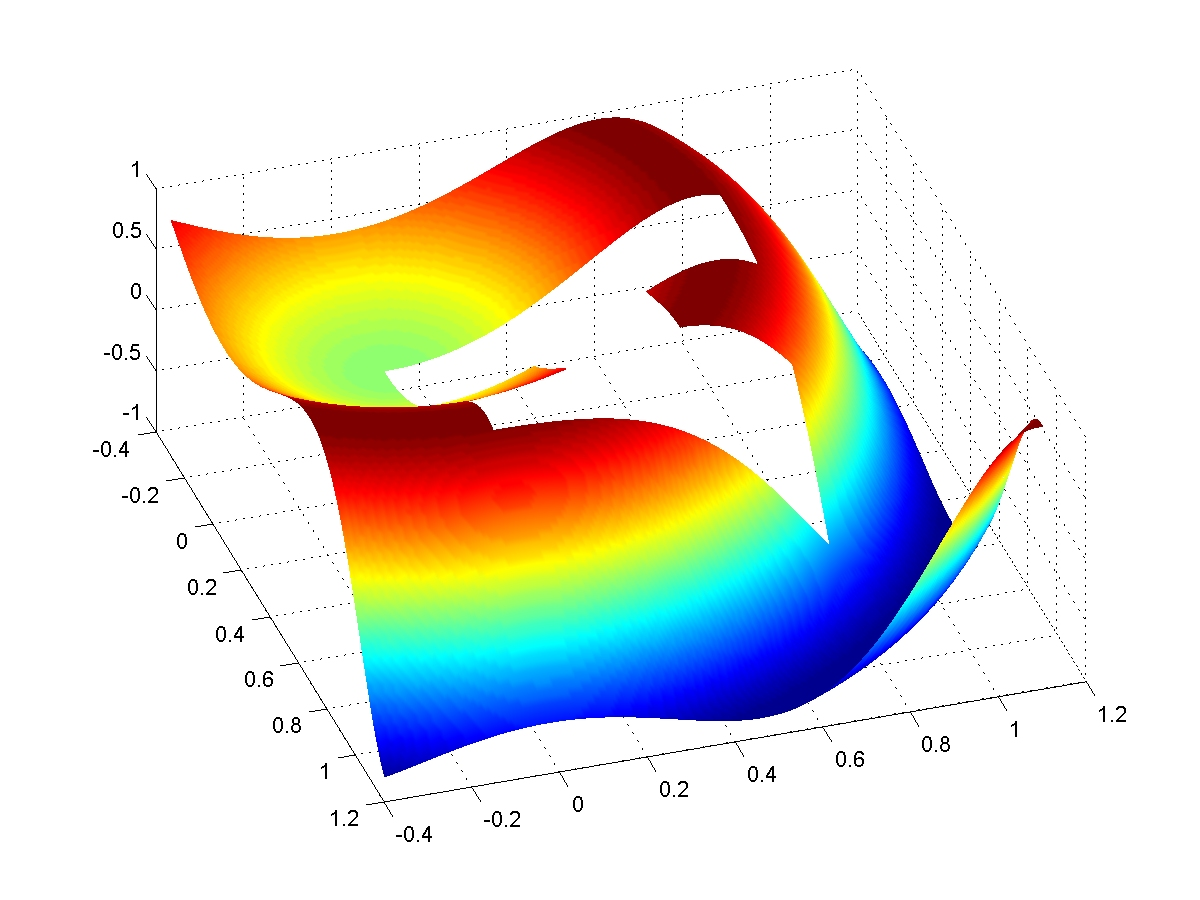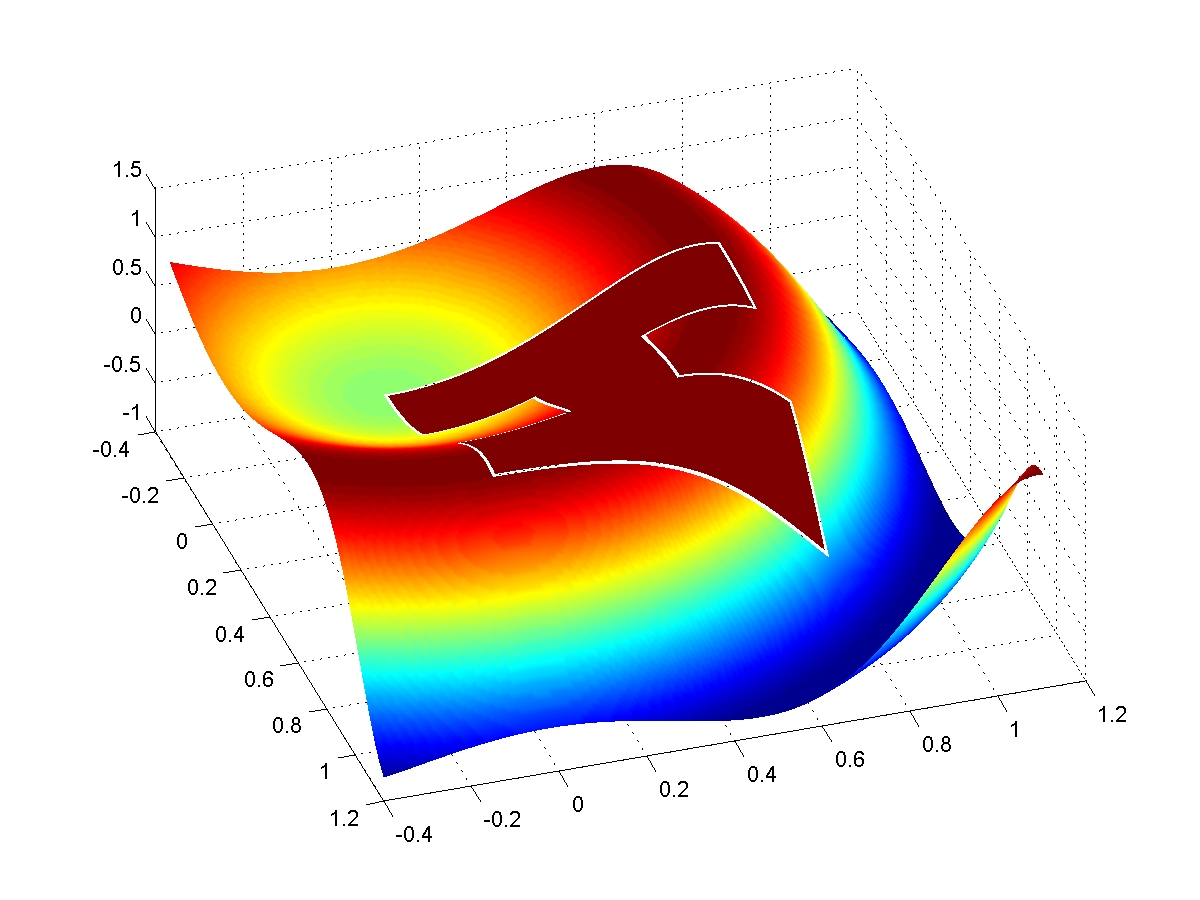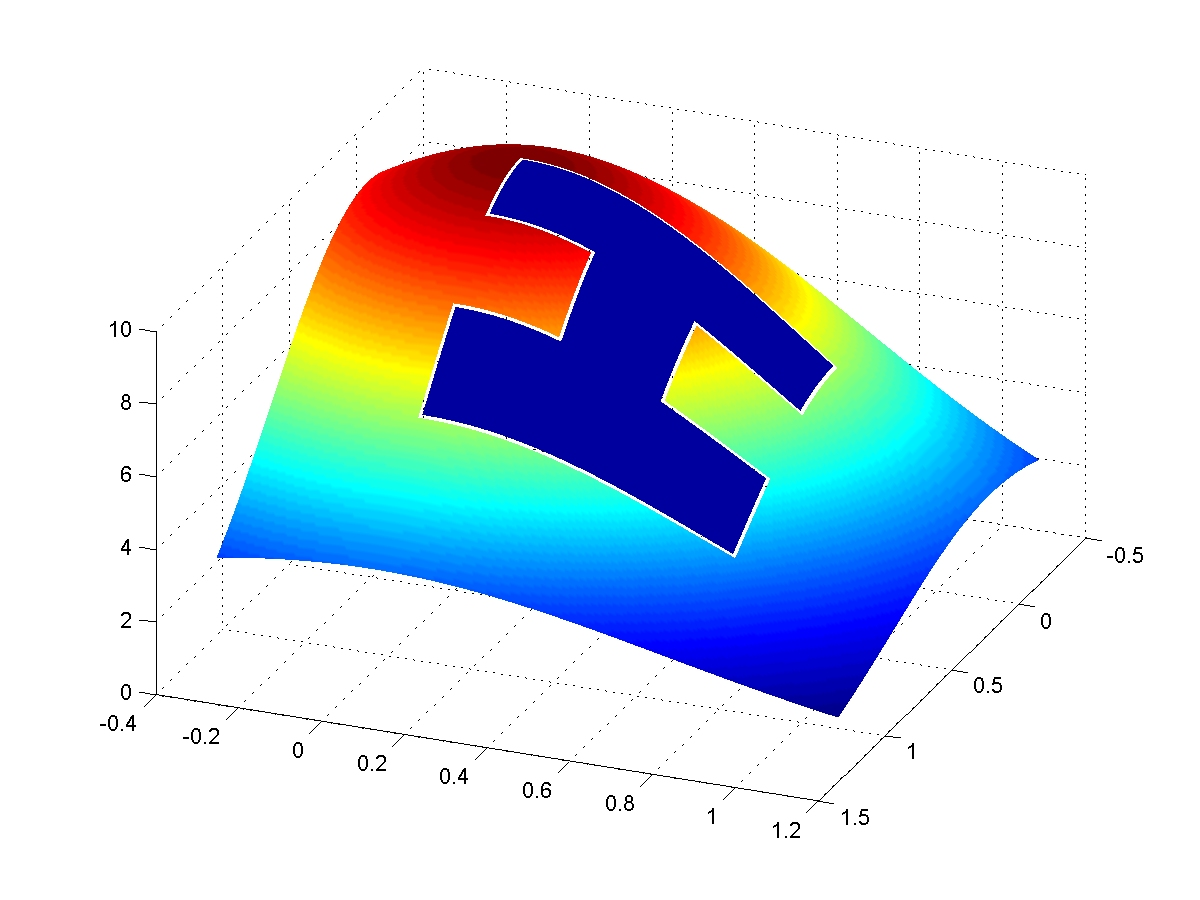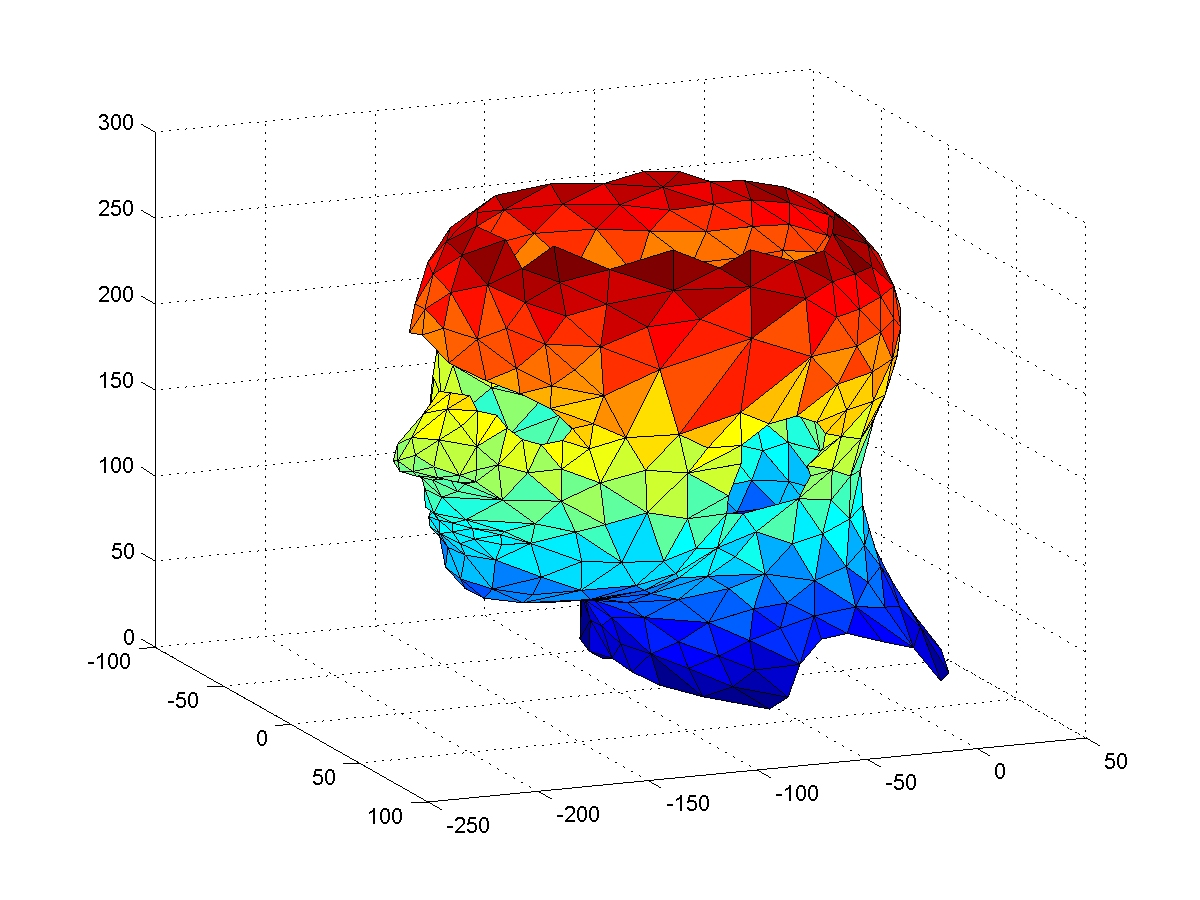# Hole Filling

All examples below are based on the results in my paper Chui, C. K. and Lai, M. J., Filling Polygonal Holes using $C^1$ Cubic Triangular Spline Patches, Computer Aided Geometric Design, vol. 17 (2000) pp. 297--307. See additional examples in this paper.

Example 1: Given a surface with a hole as shown below I used C1 quintic splines to find a surface which interpolates the values and derivatives on the boundary of the hole. The mending surface is shown.Example 2: Given a surface with a hole as shown below. I used C1 quintic splines to find a surface which interpolates the values and derivates on the boundary of the hole. The mending surface is shown in the following.Example 3: Given a surface with a hole as shown below. I used C1 quintic splines to find a surface which interpolates the values and derivates on the boundary of the hole. The mending surface is shown in the following.Example 4: Given a surface with a hole as shown below. I used C1 quintic triangulated spherical splines to find a surface which interpolates the values and derivates on the boundary of the hole. The mending surface is shown in the following. Another filling with artificial horns added is shown in the end.